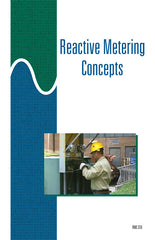#### Get the Study Guide for this videoFollow the video session-by-session with this handy book. Color illustrations throughout. \$15

Instructor Guide  available

# Reactive Metering Concepts - Instant Video

##### Summary

This 34-minute video uses the power triangle to illustrate the relationships between active power, apparent power, and reactive power. The concept of metering reactive power is explained. In addition, this unit explains how a phase-shifting device can be used to produce the phase relationships needed to meter reactive power with conventional kWh meters.

##### Training Sessions

Power Relationships – 11 minutes
Defines reactive power, and explains why reactive power consumption is measured. Using phasor diagrams, explains the relationship between active power and reactive power. Explains how net reactive current flow is determined. Explains how to determine total current flow in a circuit using the parallelogram method of phasor addition.

Determining Power in a Circuit – 13 minutes
Explains how the Pythagorean theorem and the power triangle can be used to determine power values. Explains how to determine the power factor of a circuit using mathematical functions. States the formulas that can be used to calculate active power, reactive power, and apparent power in a balanced three-phase system. Describes how reactive power can be metered using two kWh meters and a phase shifting transformer.

Reactive Metering Connections – 10 minutes
Describes how two kilowatthour meters and two phase shifting transformers may be connected to meter reactive power in a three-phase, three-wire system. Explains how the 90-degree phase shift is accomplished in a reactive meter installation.

Item Code: RMC-IV